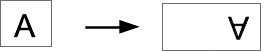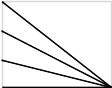# CS106A Midterm - Solution

### Instructions

• There are 7 problems to complete
• In Bluebook, "Problem 1" is these instructions and reference code
• Problems 2-8 are the actual problems
• This is a closed note exam
• The exam is 50 minutes and 100 points
• We will not grade off for syntax errors, so long as we see the correct idea
• We will not grade off if you omit the final "return" at the end of a function, so long as the code computes the correct result
• Good luck!
```# CS106A Exam Reference Reminder
# [square brackets] denote functions listed here that we have not used yet
# Exam questions will not depend on functions we have not used yet
General functions:
len() int() str() range() list() [sorted()]
String functions:
isalpha() isdigit() isspace() isupper() islower()
find() upper() lower() [strip()]
List functions:
append() extend() pop() index() [insert()]

SimpleImage:
image = SimpleImage(filename)
# create blank image
image = SimpleImage.blank(width, height)

# foreach loop
for pixel in image:

# range/y/x loop
for y in range(image.height):
for x in range(image.width):
pixel = image.get_pixel(x, y)

Canvas Draw
canvas.draw_line(x1, y1, x2, y2)
# x,y is upper left, optional color='red' parameter
canvas.draw_rect(x, y, width, height)
canvas.fill_rect(x, y, width, height)
canvas.draw_oval(x, y, width, height)
canvas.fill_oval(x, y, width, height)

Grid 2D:
grid = Grid.build([['row', '0'], ['row', '1']])
grid.width, grid.height - properties
grid.in_bounds(x, y) - True if in bounds
grid.get(x, y) - returns contents at that x,y, or None if empty
grid.set(x, y, value) - sets new value into grid at x,y

Read lines out of a text fie:
with open(filename) as f:
for line in f:
```

### 2. Short Answer (5 points)

What 3 numbers print when the caller() function runs? This code runs without error.

```def foo(x):
x = x + 2
y = 0
return x

def caller():
x = 2
y = 3
z = foo(y)
print(x, y, z)
```
```# Write 3 numbers here

```

### 3. List Ops (20 points)

Say we have a list of string id codes, and a matching list of ages. Each id code is in lowercase form and represents one person. The ids and ages lists are the same length, like this:

```ids  = ['qxj12aa', 'pr31x',  'Xxz93a', ...]
ages = [       19,      12,        21, ...]
```

So in the above example person 'qxj12aa' is 19 years old, person 'pr31x' is 12, and so on.

For this function, you are given both lists and one id, like 'Pr31X' and the question is: is this person legally able to vote? We'll say that someone can vote if their id is present in the ids list, and their age is at least 18. The ids in the list are in all lowercase form, but the id passed into the function may not be, such as 'Pr31X'. (Thanks to Brahm for creating this problem.)

```def can_vote(ids, ages, id):

```

### 4. Otter Graphics (10 points)

Given an image, create a new blank "out" image which is 2x as wide and the same height as the original. Create an upside down copy of the original image in the right half of the out image, leaving the left half of the out image untouched. Some boilerplate code is provided to start.```def right_down(filename):
image = SimpleImage(filename)

out = - your code here -

for y in range(image.height):
for x in range(image.width):
pixel = image.get_pixel(x, y)
pass

```

### 5. Drawing (10 points)

Reminder: the canvas draw_line function:

```canvas.draw_line(x1, y1, x2, y2) - draws a line from x1,y1 to x2,y2
```

As on the Quilt homework, the function will take in the x,y width,height of a figure positioned on the canvas, and draw lines within that figure.

Given int n which is 2 or more, draw n lines, each starting on the left edge and ending at the lower right corner of the figure. The first line should start at the upper left corner, the last line should start at the lower left corner, with the other lines distributed evenly.

Here is a picture showing the figure boundaries in gray with n=4 lines```def draw(canvas, x, y, width, height, n):
pass

```

### 6. Grid (20 points)

Suppose there is a grid where every square is either a bubble `'b'`, rock `'r'`, or empty `None`. Write code for a `bubble_up(grid, y)` function that tries to move every bubble in a row up one square as follows: given a grid and an in-bounds y value. Look at every square with that y. If the square contains a bubble `'b'`, and the square above it is empty, move the bubble up one square, changing its original square to be empty. Here is an example run of `bubble_up(grid, y)` for the row y=1 in Doctest format:

```>>> grid = Grid.build([[None, 'r', 'b'], ['b', 'b', 'b']])
>>> bubble_up(grid, 1)
[['b', 'r', 'b'], [None, 'b', 'b']]
```
```def bubble_up(grid, y):
pass

```

### 7. Parse1 (10 points)

Given a string s. Find the first `'+'` in s. The `'+'` is guaranteed to be followed by 2 digits, such as in 'xx+12xx'. Return the integer value of the 2 digits, 12 in this example. If there is no `'+'` in s, return -1.

```def plus(s):
pass

```

### 8. Parse2 (25 points)

Find each up-hat char `'^'` in s, using a while True/find() structure. Each `'^'` is immediately followed by a "word" made of zero or more digit or dot `'.'` chars, e.g. `'^11.2..9'`. Return a list of the digit/dot words for each `'^'`, so for example `'9bc^12xx^7.77'` returns `['12', '7.77']`

```'9bc^12xx^7.77') -> ['12', '7.77']
'^.1^.2^..' -> ['.1', '.2', '..']
'x^x^2.3x^5' -> ['', '2.3', '5']
```
```def parse_up(s):
search = 0
result = []
while True:
pass

```

## Solutions

```Solutions

2.
2 3 5

3. List Ops
def can_vote(ids, ages, id):
low = id.lower()
if low in ids:
index = ids.index(low)
# Could write a loop to search, but .index() is easier
age = ages[index]
if age >= 18:
return True
return False

4. Otter Graphics

def right_down(filename):
image = SimpleImage(filename)

out = SimpleImage.blank(image.width * 2, image.height)

for y in range(image.height):
for x in range(image.width):
pixel = image.get_pixel(x, y)
# The x,y complexity is concentrated in this line:
out_pixel = out.get_pixel(image.width + x, image.height - 1 - y)
out_pixel.red = pixel.red
out_pixel.green = pixel.green
out_pixel.blue = pixel.blue

5. Canvas Drawing

def draw(canvas, x, y, width, height, n):
for i in range(n):
y_add = i/(n - 1) * (height - 1)
# Draw from points going down left side, all to lower-right corner
canvas.draw_line(x, y + y_add, x + width - 1, y + height - 1)

6. Grid

def bubble_up(grid, y):
"""
>>> grid = Grid.build([[None, 'r', 'b'], ['b', 'b', 'b']])
>>> bubble_up(grid, 1)
[['b', 'r', 'b'], [None, 'b', 'b']]
"""
# Look at every x for the given y
for x in range(grid.width):
if grid.get(x, y) == 'b':
# Is the "up" square in bounds and empty?
# (Could use in_bounds() for this)
if y - 1 >= 0 and grid.get(x, y - 1) == None:
grid.set(x, y - 1, 'b')
grid.set(x, y, None)

7. Parse1

def plus(s):
"""
>>> plus('xx+12xx')
12
>>> plus('ab12+99z')
99
>>> plus('abc12')
-1
"""
plus = s.find('+')
if plus != -1:
substr = s[plus + 1:plus + 3]
return int(substr)
return -1

8. Parse2

def parse_up(s):
"""
>>> parse_up('9bc^12xx^7.77')
['12', '7.77']
>>> parse_up('^.1^.2^..')
['.1', '.2', '..']
>>> parse_up('x^x^2.3x^5')
['', '2.3', '5']
"""
search = 0
result = []
while True:
pass
uphat = s.find('^', search)
if uphat == -1:
break
end = uphat + 1
while end < len(s) and (s[end].isdigit() or s[end] == '.'):
end += 1
word = s[uphat + 1:end]
result.append(word)
search = end
return result
```